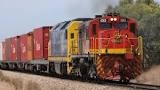# Purchase 8073

The company paid a 20% tax on the purchase of the machine in the amount of 240 €. What is the price of the machine without tax?

a =  1200 Eur

### Step-by-step explanation:

0.20a = 240

0.20·a = 240

0.2a = 240

a = 240/0.2 = 1200

a = 1200

Our simple equation calculator calculates it.Did you find an error or inaccuracy? Feel free to write us. Thank you!

Tips for related online calculators
Our percentage calculator will help you quickly calculate various typical tasks with percentages.
Do you have a linear equation or system of equations and looking for its solution? Or do you have a quadratic equation?